# Quantity of Cement and Sand Calculation in MortarReading time: 1 minute

## Quantity of Cement and Sand Calculation in Mortar

Quantity of cement mortar is required for rate analysis of brickwork and plaster or estimation of masonry work for a building or structure. Cement mortar is used in various proportions, i.e. 1:1, 1:2, 1:3, 1:4, 1:6, 1:8 etc.

### Calculation of quantity of cement mortar in brickwork and plaster:

For the calculation of cement mortar, let us assume that we use 1m3 of cement mortar. Procedure for calculation is: 1. Calculate the dry volume of materials required for 1m3 cement mortar. Considering voids in sands, we assume that materials consists of 60% voids. That is, for 1m3 of wet cement mortar, 1.6m3 of materials are required. 2. Now we calculate the volume of materials used in cement mortar based on its proportions. Let’s say, the proportion of cement and sand in mortar is 1:X, where X is the volume of sand required. Then, the volume of sand required for 1:X proportion of 1m3 cement mortar will be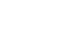3. Volume of cement will be calculated as: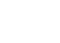Since the volume of 1 bag of cement is 0.0347 m3, so the number of bag of cement will be calculated as: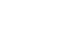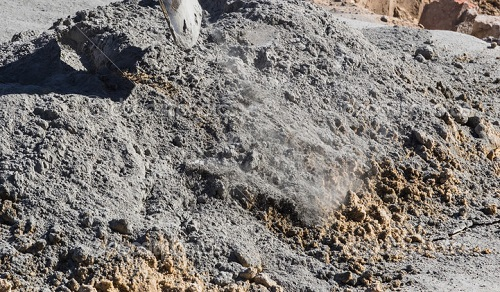Example: For cement mortar of 1:6, the quantity calculated will be as below: Sand quantity: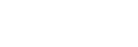Quantity of cement (in bags): Volume of cement =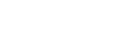There number of bags required =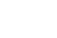= 6.58 bags.